# php中遍历数组的五种方法是什么php中遍历数组的五种方法

for循环会将控制循环次数的变量预先定义在for语句中，因此for循环语句能够按照已知的循环次数进行循环操作，适用于明确知道脚本需要运行的次数的情况。

for 循环的语法格式如下：

```for (初始化语句; 循环条件; 变量更新--自增或自减) {
语句块;
}```

```<?php
\$array= array(2,"数学","梨子",3.14,"榴莲",12);
for (\$i=0; \$i < count(\$array); \$i++) {
echo \$array[\$i] . "<br/>";
}
?>```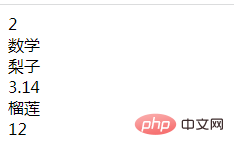foreach是专门为遍历数组而设计的语句，是遍历数组时常用的方法，在遍历数组方面提供了很大的便利；PHP5后还可以遍历对象（foreach仅能够应用于数组和对象）。

foreach语句遍历数组与数组下标无关，且可以用于不连续的索引数组和以字符串为下标的关联数组。

```<?php
\$array= array("香蕉","苹果","梨子","橙子","橘子","榴莲");
foreach (\$array as \$val){
echo "值是：" . \$val ;
echo "<br/>";
}
echo "<br/>";
foreach (\$array as \$key => \$value) {
echo "键名为：".\$key."，键值为：".\$value . "<br/>";
}
?>```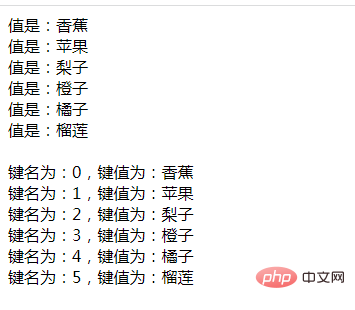each() 函数返回数组中当前的键值并将数组指针向前移动。在执行 each() 之后，数组指针将停留在数组中的下一个元素或者当碰到数组结尾时停留在最后一个元素。

while循环+each() 函数+ list() 函数可以遍历数组，下面通过代码示例来看看：

```<?php
\$array= array("香蕉","苹果","梨子","橙子","橘子","榴莲");
while( list(\$key, \$val) = each(\$array) ) {
echo "\$key => \$val<br>";
}
?>```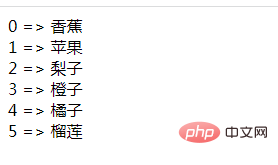```<?php
\$arr = ['a', 'b', 'c'];
var_dump(\$arr);
\$arr = array_map(function (\$item) {
return \$item . '_i';
}, \$arr);
var_dump(\$arr);
?>```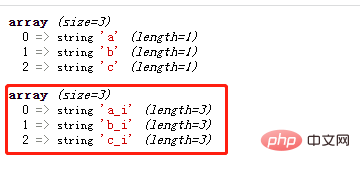```<?php
\$arr_1 = ['a', 'b', 'c'];
\$arr_2 = ['你', '好', '吗', '原', '罪'];
// \$arr_n = [...];
\$arr = array_map(function (\$item_1, \$item_2) {
return \$item_1 . '_' . \$item_2 . '_i';
}, \$arr_1, \$arr_2);

var_dump(\$arr); // ['a_你_i', 'b_好_i', 'c_吗_i', '_原_i', '_罪_i']
?>```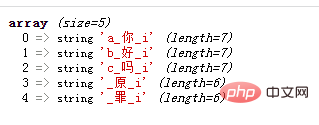```<?php
\$arr = ['a', 'b', 'c'];
array_walk(\$arr, function (&\$item) {
\$item = \$item . '_i';
});

var_dump(\$arr);
?>```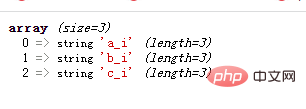• 相关标签：php php数组
• 相关文章

相关视频